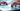## Policy invariance under reward transformations: Theory and application to reward shaping

This paper investigates conditions under which modifications to the reward function of a Markov decision process preserve the optimal policy. It is shown that, besides the positive linear transformation familiar from utility theory, one can add a reward for transitions between states that is expressible as the difference in value of an arbitrary potential function […]## Approximate planning in large POMDPs via reusable trajectories

We consider the problem of reliably choosing a near-best strategy from a restricted class of strategies › in a partially observable Markov decision process (POMDP). In particular, we are interested in what might be considered the sample complexity — that is, the amount of data or experience we must generate in the POMDP in order […]## Policy search via density estimation

We propose a new approach to the problem of searching a space of stochastic controllers for a Markov decision process (MDP) or a partially observable Markov decision process (POMDP). Following several other authors, our approach is based on searching in parameterized families of policies (for example, via gradient descent) to optimize solution quality. However, rather […]## Approximate inference algorithms for two-layer Bayesian networks

We present a class of approximate inference algorithms for graphical models of the QMR-DT type. We give convergence rates for these algorithms and for the Jaakkola and Jordan (1999) algorithm, and verify these theoretical predictions empirically. We also present empirical results on the difficult QMR-DT network problem, obtaining performance of the new algorithms roughly comparable […]## Algorithms for inverse reinforcement learning

This paper addresses the problem of inverse reinforcement learning (IRL) in Markov decision processes, that is, the problem of extracting a reward function given observed, optimal behavior. IRL may be useful for apprenticeship learning to acquire skilled behavior, and for ascertaining the reward function being optimized by a natural system. We first characterize the set […]## PEGASUS: A policy search method for large MDPs and POMDPs

We propose a new approach to the problem of searching a space of policies for a Markov decision process (MDP) or a partially observable Markov decision process (POMDP), given a model. Our approach is based on the following observation: Any (PO)MDP can be transformed into an “equivalent” POMDP in which all state transitions (given the […]## Data-Intensive Question Answering

Microsoft Research Redmond participated for the first time in TREC this year, focusing on the question answering track. There is a separate report in this volume on the Microsoft Research Cambridge submissions for the filtering and Web tracks (Robertson et al., 2002). We have been exploring data-driven techniques for Web question answering, and modified our […]## Convergence rates of the Voting Gibbs classifier, with application to Bayesian feature selection

The Gibbs classifier is a simple approximation to the Bayesian optimal classifier in which one samples from the posterior for the parameter », and then classifies using the single classifier indexed by that parameter vector. In this paper, we study the Voting Gibbs classifier, which is the extension of this scheme to the full Monte […]## Stable algorithms for link analysis

The Kleinberg HITS and the Google PageRank algorithms are eigenvector methods for identifying “authoritative” or “influential” articles, given hyperlink or citation information. That such algorithms should give reliable or consistent answers is surely a desideratum, and in , we analyzed when they can be expected to give stable rankings under small perturbations to the linkage […]## Link analysis, eigenvectors, and stability

The HITS and the PageRank algorithms are eigenvector methods for identifying “authoritative” or “influential” articles, given hyperlink or citation information. That such algorithms should give consistent answers is surely a desideratum, and in this paper, we address the question of when they can be expected to give stable rankings under small perturbations to the hyperlink […]## Latent Dirichlet Allocation

We describe latent Dirichlet allocation (LDA), a generative probabilistic model for collections of discrete data such as text corpora. LDA is a three-level hierarchical Bayesian model, in which each item of a collection is modeled as a finite mixture over an underlying set of topics. Each topic is, in turn, modeled as an infinite mixture […]## On Spectral Clustering: Analysis and an algorithm

Despite many empirical successes of spectral clustering methods— algorithms that cluster points using eigenvectors of matrices derived from the data—there are several unresolved issues. First, there are a wide variety of algorithms that use the eigenvectors in slightly different ways. Second, many of these algorithms have no proof that they will actually compute a reasonable […]

© 2022 Andrew Ng